# Problem Solving with Computer

By Bipin Tiwari

Problem Solving is a scientific technique to discover and implement the answer to a problem. The computer is the symbol manipulating device that follows the set of commands known as program.

### Program:

Program is the set of instructions which is run by the computer to perform specific task. The task of developing program is called programming.

### Problem Solving Technique:

Sometimes it is not sufficient just to cope with problems. We have to solve that problems. Most people are involving to solve the problem. These problem are occur while performing small task or making small decision. So, Here are the some basic steps to solve the problems

Step 1: Identify and Define Problem

Explain you problem clearly as possible as you can.

Step 2: Generate Possible Solutions

• List out all the solution that you find. Don’t focus on the quality of the solution
• Generate the maximum number of solution as you can without considering the quality of the solution

Step 3: Evaluate Alternatives

After generating the maximum solution, Remove the undesired solutions.

Step 4: Decide a Solution

After filtering all the solution, you have the best solution only. Then choose on of the best solution and make a decision to make it as a perfect solution.

Step 5: Implement a Solution:

After getting the best solution, Implement that solution to solve a problem.

Step 6: Evaluate the result

After implementing a best solution, Evaluate how much you solution solve the problem. If your solution will not solve the problem then you can again start with Step 2.

## Algorithm:

Algorithm is the set of rules that define how particular problem can be solved in finite number of steps. Any good algorithm must have following characteristics

1. Input: Specify and require input
2. Output: Solution of any problem
3. Definite: Solution must be clearly defined
4. Effective
5. Finite: Steps must be finite
6. Correct: Correct output must be generated

1. It is the way to sole a problem step-wise so it is easy to understand.
2. It uses definite procedure.
3. It is not dependent with any programming language.
4. Each step has it own meaning so it is easy to debug

1. It is time consuming
2. Difficult to show branching and looping statement
3. Large problems are difficult to implement

## Flowchart:

The solution of any problem in picture form is called flowchart. It is the one of the most important technique to depict an algorithm.

1. Easier to understand
2. Helps to understand logic of problem
3. Easy to draw flowchart in any software like MS-Word
4. Complex problem can be represent using less symbols
5. It is the way to documenting any problem
6. Helps in debugging process

1. For any change, Flowchart have to redrawn
2. Showing many looping and branching become complex
3. Modification of flowchart is time consuming

### Symbol Used in Flowchart:

 Symbol Name Description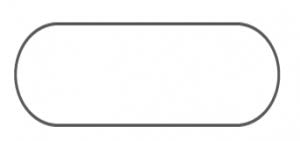Terminal Terminal represent start and end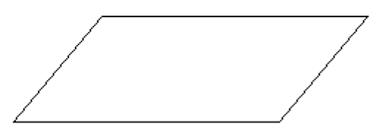Input / Output Used for input (reading) and output (printing) operation.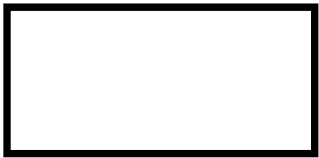Processing Used for data manipulation and data operations.Arrow Used to represent flow of operations.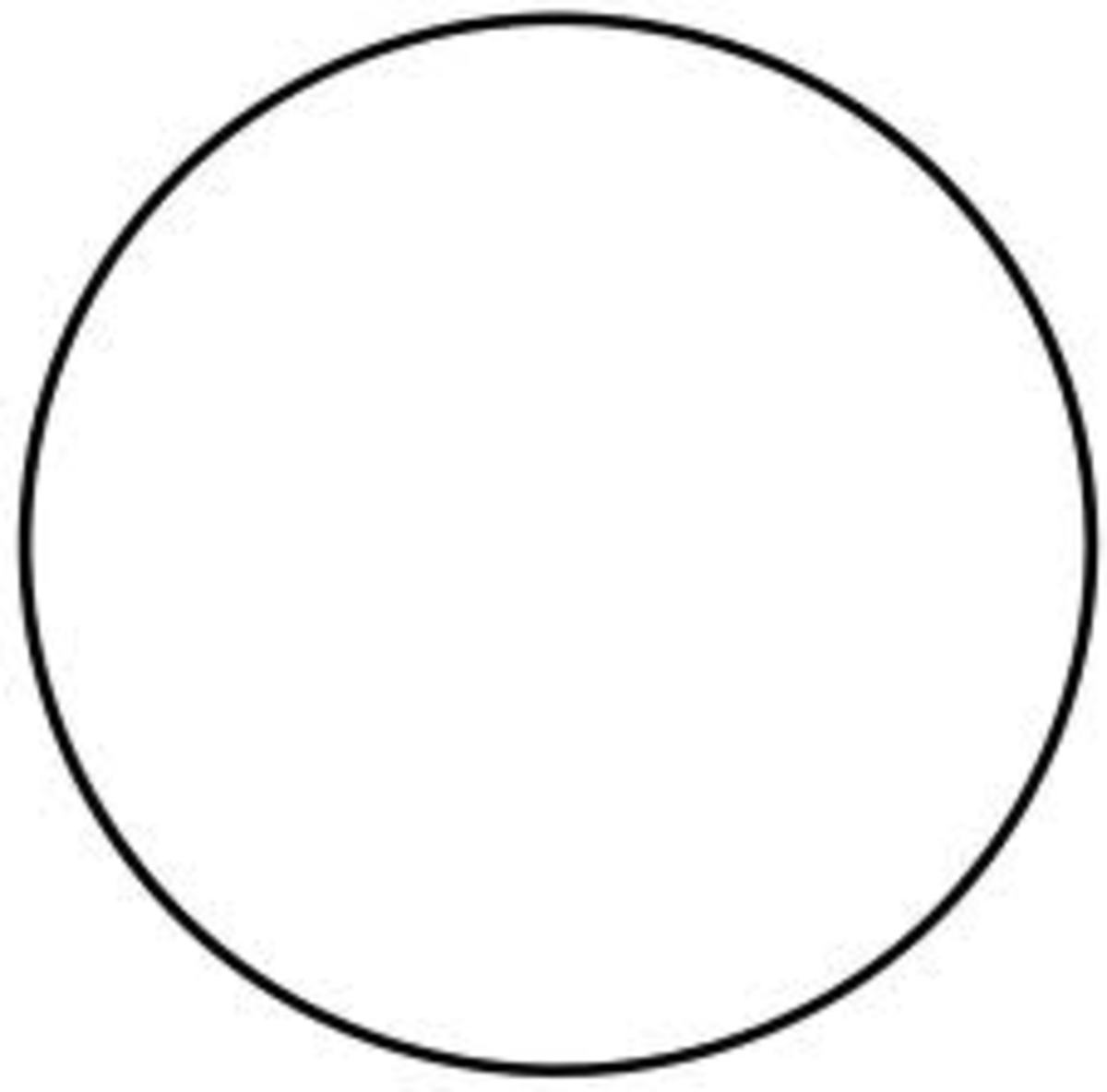Connector Used to connect different flow of lines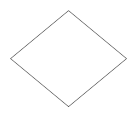Decision Used to make decision

Example: Algorithm and Flowchart to check odd or even

Solution:

Algorithm:

Step 1: Start
Step 2: Declare a variable x
Step 3: Take a input from user and  store in x
Step 4: IF x % 2 == 0 THEN
PRINT Even
ELSE
PRINT Odd
Step 5: End

Flowchart: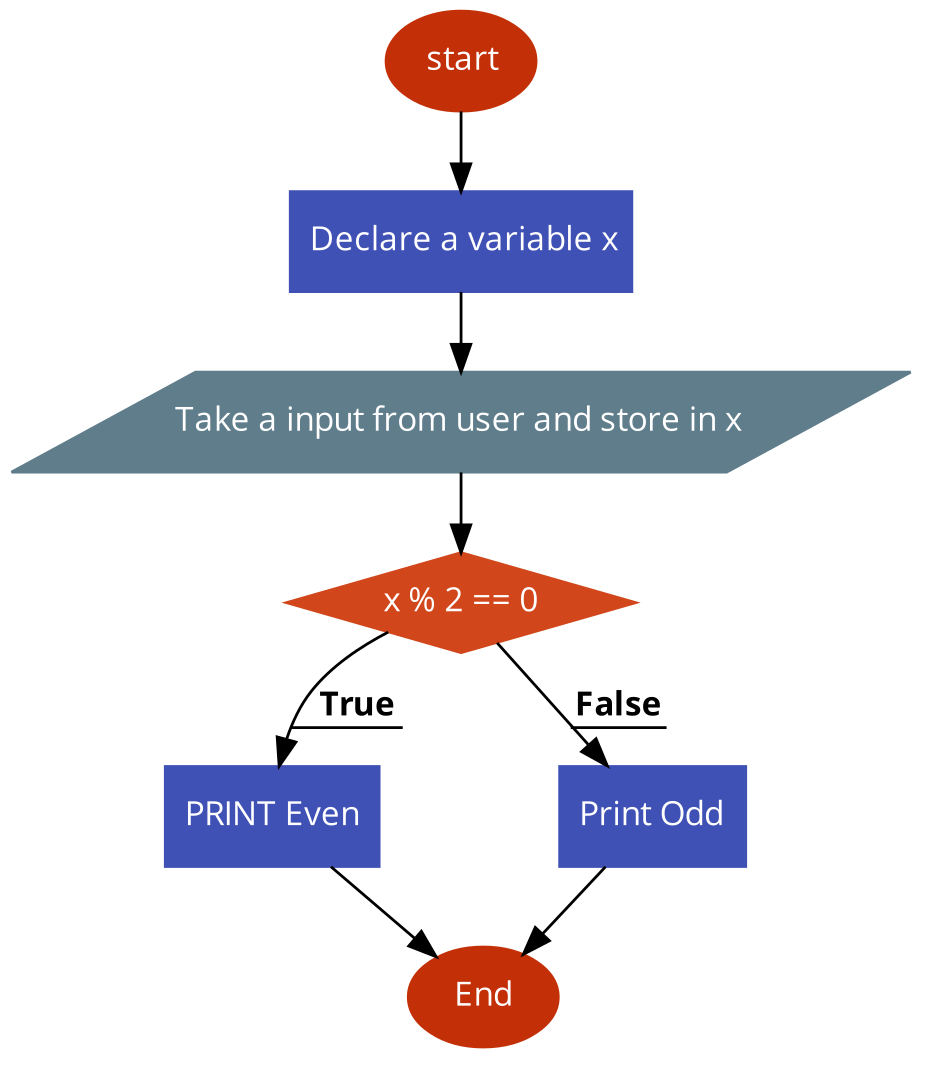## Coding, Compiling and Execution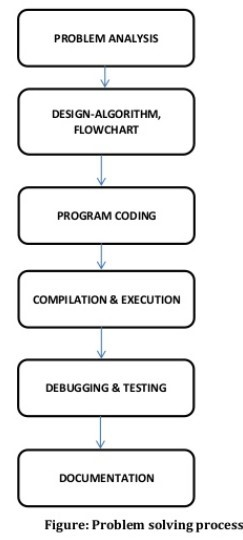Important Questions